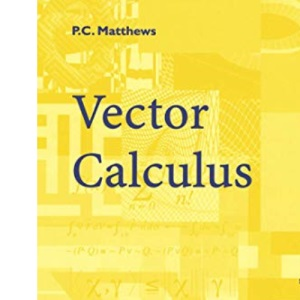30 Day Refund Guarantee# Vector Calculus (Springer Undergraduate Mathematics Series)

by PaulC.Matthews (Author)

##### Synopsis

Vector calculus is the fundamental language of mathematical physics. It pro- vides a way to describe physical quantities in three-dimensional space and the way in which these quantities vary. Many topics in the physical sciences can be analysed mathematically using the techniques of vector calculus. These top- ics include fluid dynamics, solid mechanics and electromagnetism, all of which involve a description of vector and scalar quantities in three dimensions. This book assumes no previous knowledge of vectors. However, it is assumed that the reader has a knowledge of basic calculus, including differentiation, integration and partial differentiation. Some knowledge of linear algebra is also required, particularly the concepts of matrices and determinants. The book is designed to be self-contained, so that it is suitable for a pro- gramme of individual study. Each of the eight chapters introduces a new topic, and to facilitate understanding of the material, frequent reference is made to physical applications. The physical nature of the subject is clarified with over sixty diagrams, which provide an important aid to the comprehension of the new concepts. Following the introduction of each new topic, worked examples are provided. It is essential that these are studied carefully, so that a full un- derstanding is developed before moving ahead. Like much of mathematics, each section of the book is built on the foundations laid in the earlier sections and chapters.

### \$54.85

Quantity

20+ in stock

Format: Paperback
Pages: 200
Edition: 1st ed. 1998. Corr. 3rd printing
Publisher: Springer
Published: 14 Jan 1998

ISBN 10: 3540761802
ISBN 13: 9783540761808
Book Overview: Springer Book Archives

##### Media Reviews

P.C. Matthews

Vector Calculus

Written for undergraduate students in mathematics, the book covers the material in a comprehensive but concise manner, combining mathematical rigor with physical insight. There are many diagrams to illustrate the physical meaning of the mathematical concepts, which essential for a full understanding of the subject. - ZENTRALBLATT MATH

×